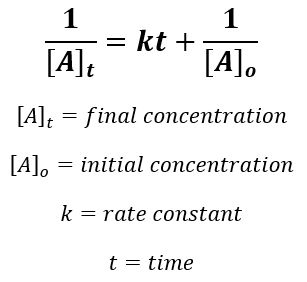# Problem: The reaction X → products is second order in X and has a rate constant of 0.035 M-1s-1.  If a reaction mixture is initially 0.55 M in X, what is the concentration of X after 151 seconds?

###### FREE Expert Solution

For a second order reaction we’re going to use the following equation:Given:

Initial concentration of X = 0.55 M
t = 151 s
k = 0.035 M-1 s-1

Calculate final concentration of X:

89% (23 ratings)###### Problem Details

The reaction X → products is second order in X and has a rate constant of 0.035 M-1s-1.  If a reaction mixture is initially 0.55 M in X, what is the concentration of X after 151 seconds?